AP Board 9th Class Physical Science Important Questions Chapter 6 Chemical Reactions and Equations

AP State Syllabus AP Board 9th Class Physical Science Important Questions Chapter 6 Chemical Reactions and Equations

AP State Syllabus 9th Class Physical Science Important Questions 6th Lesson Chemical Reactions and Equations

9th Class Physical Science6th Lesson Chemical Reactions and Equations 1 Mark Important Questions and Answers

Question 1.
Fe2O3 + 2 Al → Al2O3 + 2Fe
Name the compound which is oxidized in the above reaction.
In the reaction Fe2O3 + 2Al → Al2O3 + 2Fe,
Aluminium (Al) is oxidised and formed as Al2O3.

Question 2.
Give an example for displacement reaction.
1) In displacement reaction one element displaces another element from its compound and takes its place there in.

2) Eg : Zinc pieces react with dilute hydrochloric acid and liberate Hydrogen gas.
Zn(s)+ 2HCl(aq) → ZnCl2(aq) + H2(g)Question 3.
A shiny brown coloured element ‘X’ on heating in air becomes black in colour. Assume and write the name of the element ‘X’ and also predict the substance formed black in colour.
Element X – Copper.
Black substance – Copper Oxide

Question 4.
Iron gets rust but Gold doesn’t, why?
Gold does not oxidized.
(OR)
Gold is least reactive metal.

Question 5.
What happens if iron articles are exposed to moist air? Write the chemical equation to represent that reaction.
Rusting takes place on iron articles when they are exposed to moist air.
2Fe(s) + O2(g) + 4H+(aq) → 2Fe2+(aq) + 2H2O(l)

Question 6.
On adding dilute hydrochloric acid to copper oxide powder, the solution formed is blue green. Write the new compound formed.
Copper oxide reacts with hydrochloric acid and forms copper chloride and water. Copper chloride is in blue green colour.
CuO + 2 HCl → CuCl2 + H2O

Question 7.
Write the equation for the chemical decomposition reaction of silver chloride in the presence of sunlight.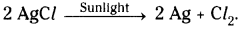Question 8.
Balance the following chemical equation.
Na + H2O → NaOH + H2
2Na + 2H2O → 2NaOH + H2Question 9.
If you keep an iron piece in solid state CuSO4 crystals, does it get any reaction? Guess the reason.
Reaction will not takes place if an iron piece is placed in solid state CuSO4 crystals because there will not exist separate Cu+2, SO4-2 ions in CuSO4 Crystals. In aqueous solution, they exists. So iron can not displace copper.

Question 10.
What is a chemical equation?
Chemical Equation :
Describing a chemical reaction using least possible words or symbols is called a chemical equation.
Ex : CaO + H2O → Ca(OH)2

Question 11.
What are “Reactants” and “Products”?
1) Reactants :
The substances which undergo chemical change in the reaction are called ‘Reactants’.

2) Products:
The new substances formed in a chemical reaction are called ‘Products’.
Ex : Zn + 2HCl → ZnCl2 + H2
3) In above reaction Zn and 2HCl are called reactants, ZnCl2 and H2 are called products.

Question 12.
What is a balanced chemical equation?
Balanced Chemical Equation :
A chemical reaction in which the number of atoms of different elements on the reactants side (left side) are same as those on product side (right side) is called a balanced chemical equation.
Ex : Mg + O2 → MgO (Unbalanced chemical equation)
2 Mg + O2 → MgO (Balanced chemical equation)

Question 13.
What is chemical change?
Chemical Change :
The process in which the change is affecting the identity of a molecule by a change in chemical composition is called a “chemical change”.

Question 14.
What is physical change?
Physical Change: The process where a change is occurring only in physical properties, without affecting the identity of the molecules is called a “physical change”.

Question 15.
What is the meaning of “Exo”, “Endo” and “Thermo”?
The term ‘Exo’ means outside, ‘Endo’ means inside and ‘Thermo’ means heat.Question 16.
What is oxidation? Give examples.
Oxidation is a reaction that involves the addition of oxygen or loss of hydrogen or electrons.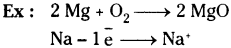Question 17.
What is a reduction? Give examples.
The process in which a substance loses oxygen or gains hydrogen or electrons is known as reduction.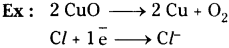Question 18.
What is corrosion?
Corrosion :
When some metals are exposed to moisture, acids etc. they tarnish due to the formation of respective metal oxide on their surface. This process is called “corrosion”.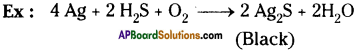Question 19.
What is “galvanizing”?
It is a method of protecting iron from rusting by coating them a thin layer of zinc.

Question 20.
What is combustion?
The process of burning a substance in the presence of oxygen is called “combustion”.

Question 21.
What do you mean by “rancidity”?
Food materials containing fat / oil are exposed to air, for a long time they react with atmospheric oxygen and it is responsible for spoiling of food. This process is called “rancidity”.

Question 22.
How do you know that respiration is an exothermic reaction?
During the respiration reaction energy releases, so it is an exothermic reaction.

Question 23.
What will happen in chemical reaction?
New substances are formed in chemical reaction.Question 24.
What is precipitate?
A precipitate is a solid product’ which separates out from the solution during a chemical reaction.

Question 25.
How does precipitation reaction occur? Explain with an example.

1. Prepare lead nitrate and potassium iodide solutions in separate test tubes.
2. Mix the two solutions.
3. A yellow colour substance which is insoluble in water, is formed. This insoluble substance is known as precipitate. The precipitate here in this reaction is lead iodide.
Pb(NO3)2 + 2KI → Pbl2 + 2KNO3

Question 26.
What is a balanced chemical equation?
A balanced chemical equation has an equal number of atoms of different elements in the reactants and products.

Question 27.
What is an unbalanced equation?
An unbalanced chemical equation has an unequal number of atoms of one or more elements in the reactants and products.

Question 28.
Why do the smell and taste of food items change?
When fats and oils are oxidized they become rancid. So their smell and taste change.

Question 29.
“Freshly cut apple turning brown, the iron articles shiny when new, but gradually become reddish brown when left for sometime ………”. How do these changes occur?
Oxygen molecules interact with different substance from metal to living tissue which may come into contact with it. The above changes occur. These are all the examples of the process of oxidation.Question 30.
What are antioxidants?
Antioxidants :
The spoilage of food can be prevented by adding preservatives which prevent oxidation. The substances which prevent oxidation are called antioxidants.
(OR)
The substances which prevent oxidation added to food containing fats and oil are called antioxidants.

Question 31.
Complete the following reaction.
Pb(NO3)2 + 2 KI → …………….. + …………………
Pb(NO3)2 + 2 KI → Pbl2 + 2KNO3

Question 32.
If iron nail is dipped in copper sulphate solution, after sometime copper will be formed. Write the chemical equation for this reaction.
Fe + CuSO4 → FeSO4 + Cu

Question 33.
Which metal is used in the manufacture of Diwali crackers?
The metal used in manufacture of Diwali crackers is Magnesium.

Question 34.
What are new substances formed due to decomposition of lead nitrate?
The new substances formed are lead oxide, nitrogen dioxide and oxygen.Question 35.
Balance the following chemical equation. C2H6 + O2 → CO2 + H2O
2C2H6 + 7O2 → 4CO2 + 6H2O

Question 36.
NH4Cl → NH3 + HCl. Which type of reaction is this?
This is decomposition reaction.

Question 37.
Which chemical reaction is involved in the corrosion of iron?
The chemical reaction involved in corrosion of iron is oxidation reaction.

Question 38.
Which metal is used for wrapping food material? Why?
Aluminium is used to wrap food material because it reacts with oxygen and forms a protective layer of aluminium oxide which prevents further oxidation.

Question 39.
Write states of the reactants and products and also write conditions required for the completion of reaction.
2H2O → 2H2 + O2
H2O is in liquid state. H2 and 02 are in gaseous state.
The reaction is carried out in the presence of electricity.

Question 40.
Give an example for chemical combination reaction where only elements take part.
2 H2 + O2 → 2H2O
Here hydrogen and oxygen both are elements.Question 41.
Give an example for chemical combination where element and compound take part in the reaction.
2 NaN02 + O2 → 2 NaNO3
Here O2 is element and NaNO2 is compound.

Question 42.
Give an example where two compounds combine together to form a compound.
CaO + H2O → Ca(OH)2
Here both calcium oxide, water are compounds which combine together to form calcium hydroxide.

Question 43.
Before burning in air, why is Mg ribbon cleaned by rubbing with a sand paper?
To remove the protection layer of basic magnesium carbonate from the surface of magnesium

Question 44.
X Pb(NO3)2 → Y PbO + Z NO2 + W O2.
If the equation is a balanced equation, what are values of coefficients X, Y, Z and W?
The balanced equation is 2 Pb(NO3)2 → 2 PbO + 4 NO2 + O2.
∴ The values are X = 2,Y = 2, Z = 4 and W = 1.

Question 45.
Why does a layer of zinc prevent rusting of iron?
Zinc reacts with oxygen and forms layer of zinc oxide which prevents further oxidation. So a layer of zinc protects iron from rusting.

Question 46.
Why does jewellery made of gold not rust?
Gold does not react with air because it has least reactivity. So gold jewellery does not rust.

Question 47.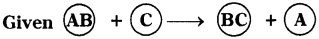What type of reaction is this? Which element between A and C is more reactive? Why?
This reaction is chemical displacement. ‘C’ is more reactive than ‘A’. So C displaces ‘A’ from AB and occupies its place.

Question 48.
Given AB + CD → AD + CB. What type of chemical reaction is this? What is the condition which makes the reaction possible?
This is double displacement reaction. This reaction can be possible if ionisation of both the salt solutions are possible.
i.e., AB → A+ + B
CD → C+ + D

Question 49.
An iron nail is completely immersed in a test tube containing oil. Do you observe any rust on the iron nail? If not, why?
No, I do not observe any rust because both air and moisture are required for rusting of iron.Question 50.
How can we prevent rusting of iron?
Rusting of iron can be prevented or at least minimised by shielding the metal surface from oxygen and moisture. It can be prevented by painting, oiling, greasing, galvanizing, chrome plating or making alloys.

Question 51.
Suggest few methods to avoid corrosion.
Some methods to avoid corrosion

1. Painting, oiling, greasing on the surfaces of the metals.
2. Galvanizing the metal with thin layer of zinc.
3. Making alloy metals prevents corrosion,
eg : brass, bronze and steel.

Question 52.
Some metals react with oxygen to form their oxides. It is serious problem. Give some examples for oxidation of metals and write balanced equations.
1) Iron reacts with oxygen and forms iron oxide.
4Fe + 3O2 → 2Fe2O3.

2) Copper reacts with oxygen and forms copper oxide.
2Cu + O2 → 2CuO

3) Silver tarnishes when reacts with hydrogen sulphide and oxygen
4Ag + 2H2S + O2 → 2Ag2S + 2H2O

Question 53.
Steel, PVC and CPVC pipes are suitable/suggestable for water supply.
Reasons:

1. They are not oxidised in moisture.
2. They do not form oxides.
3. They are not corroded.
4. So, they are durable and safe to use in supply of water.

Question 54.
Which pipes are used by you for water supply to your house?

1. PVC, CPVC and steel pipes are used for water supply in my house.
2. Because they do not corrode.

Question 55.
List of metals are given below. Classify them into corroded and non-corroded metals. Aluminium, Silver, Iroh, Copper, Gold, Tin, Tungsten, Platinum.
Corroded metals :
Aluminium, Silver, Iron, Copper

Non-corroded metals :
Gold, Tin, Tungsten, Platinum.

9th Class Physical Science6th Lesson Chemical Reactions and Equations 2 Marks Important Questions and Answers

Question 1.
A light yellow coloured compound ‘X’ is exposed to sunlight for some time. It is turned into gray coloured material. What is the name of ‘X’? Predict the type of chemical reaction occured in it.
1) The name of the compound ‘X’ is Silver Bromide (AgBr).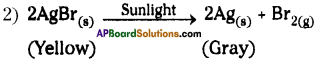This is a decomposition reaction and also it occurs in the presence of sunlight. Hence, it is a photo chemical reaction.

Question 2.
Oil and fat containing food item packets are flushed with Nitrogen gas. Why?
By stopping the oxidation it prevents the rancidity of food material.Question 3.
N2(g) + O2(g) + heat → 2NO(g)
What information do you get from the above equation? Comment.

• Nitrogen gas reacts with oxygen gas in the presence of heat energy and forms nitric oxide gas.
• It is an endothermic reaction.
• This is an example for chemical combination reaction.
• In this reaction, the reactants are gases and products are also gases.

Question 4.
Write an activity about how you conduct an experiment to show that more reactive metals replace less reactive metals from their compounds.

• Take two iron nails and clean then by rubbing with sand paper.
• Take two test tubes and mark them ‘A’ and ‘B’.
• Pour copper sulphate solution in the test tube ‘A’ and Zinc Chloride solution in the test tube ‘B’.
• Dip iron nails in both test tubes.
• Keep them without disturbing for 20 min.

Observation :

1. The nail which is dipped in Copper Sulphate solution becomes brownish colour.
2. The nail which is dipped in Zinc Chloride solution doesn’t change.

Reaction :
In test tube ‘A’: CuSO4 + Fe → FeSO4 + Cu
In test tube ‘B’: ZnCl2 + Fe → No reaction.

Conclusion :

1. Iron is more reactive than copper. So it displaces copper from Copper Sulphate solution.
2. Iron is less reactive than zinc. So, it doesn’t displace zinc from ZnClr

Question 5.
i) CaCO3(s) → CaO(s) + CO3(g)
ii) 2Ag Br(s) → 2Ag(s) + Br2(g)
Mention the types of reactions to which the above equations belong. Also mention which of them is a photochemical reaction.
1) i) CaCO3(s) → CaO(s) + CO2(g). It is a chemical decomposition reaction.
ii) 2Ag Br(s) → 2Ag(s) + Br2(g). It is also a chemical decomposition reaction.

2) 2Ag Br(s) → 2Ag(s) + Br2(g). It is a photochemical reaction. Because, this reaction
takes place in the presence of sunlight only.

Question 6.
Write the products of given reactions, if any. Give reason.
FeCl2 + Zn →
ZnCl2 + Fe →
FeCl2 + Zn → ZnCl2 + Fe (Displacement reaction)
ZnCl2 + Fe → No reaction. (Low reactive metals cannot displace high reactive metals)Question 7.
Balance the following chemical equations:
i) Na + H2O → NaOH + H2
ii) K2CO3 + HCl → KCl + H2O + CO2
i) 2Na + 2H2O → 2NaOH + H2
ii) K2CO3 + 2HCl → 2KCl + H2O + CO2

Question 8.
Observe the following balanced chemical equation and answer the given questions.
C3H8(g) + 5O2(g) → 3CO2(g) + 4H2O(g)
i) How many molecules of Oxygen are involved in this chemical reaction?
ii) How many moles of Propane are required to get 20 moles of Water?
i) In this chemical reaction five molecules of oxygen are involved.
ii) Five moles of propane are required to get 20 moles of water.

Question 9.
What do you do to prevent rusting of copper and silver articles?
I can follow some rules given below to prevent rusting of copper and silver articles.

1. Shielding the metal surface from oxygen and moisture.
2. By painting.
3. By oiling, greasing.
4. By galvanizing, chrome plating.
5. By making alloys.

Question 10.
What are the important characteristics of chemical reactions?
The important characteristics of chemical reactions are

1. Evolution of a gas
2. Formation of a precipitate
3. Change in colour
4. Change in temperature
5. Change in state

Question 11.
What symbols do we use to indicate the physical state of reactants and products in an equation?

1. Solid state is indicated by the symbol (s)
2. Liquid state is indicated by the symbol (l)
3. Gaseous state is indicated by the symbol (g)
4. Aqueous solution is indicated by the symbol (aq)

Question 12.
(OR)

1. Physical state,
2. Heat changes (exothermic and endothermic reactions),
3. Gas evolved,
4. Precipitate formed.Question 13.
Commemt on “C(s) + O2(g) → CO2(g) + Heat” equation.

• The burning of carbon in oxygen is an exothermic reaction because heat is evolved in this reaction.
• An exothermic reaction is indicated by writing + Heat or + Heat energy or just + Energy on the products side of an equation.

Question 14.
Comment on “N2(g) + O2(g) + Heat → 2 NO(g)” equation.

• The reaction between nitrogen and oxygen to form nitric oxide is an endothermic reaction because heat is absorbed in this reaction.
• An endotheumic reaction is usually indicated by writing + Heat or + Heat energy or just”+ Energy” on the reactants side of an equation.

Question 15.
Balance the following equations.
1) Na + O2 → Na2O
2) H2O2 → H2O + O2
3) Mg(OH)2 + HCl → MgCl2 + H2O
4) Fe + O2 → Fe2O2

1. 4 Na + O2 → 2 Na2O
2. 2 H2O2 → 2 H2O + O2
3. Mg(OH)2 + 2 HCl → MgCl2 + 2 H2O
4. 4 Fe + 3 O2 → 2 Fe2O3

Question 16.
2 Cu + O2 → 2 CuO
What information do you get from above equation?
The above equation tells us that,

1. Copper reacts with oxygen to form copper oxide.
2. The formula of copper oxide is CuO and that of oxygen is O2.
3. 2 moles of copper atoms react with 1 mole of oxygen molecules (O2) to produce 2 moles of copper oxide (CuO).

Question 17.
Write examples for oxidation reaction.
Oxidation :
It is a reaction involving addition of oxygen or removal of hydrogen from a substance.
1) Magnesium reacts with oxygen to form magnesium oxide.
2 Mg + O2 → 2 MgO

2) Copper reacts with oxygen to form copper oxide.
2 Cu + O2 → 2 CuO

3) Iron reacts with oxygen to form ferric oxide.
4 Fe + 3 O2 → 2Fe2O3Question 18.
Write examples for reduction reaction.
Reduction :
It is a reaction involving addition of hydrogen or removal of oxygen from a substance.
1) Nitrogen gas reacts with hydrogen gas to produce Ammonia.
N2 + 3H2 → 2NH3

2) Oil reacts with hydrogen to form fat.
Oil + H2 → Fat

Question 19.
Write the examples for corrosion reaction.
1) The black coatings on silver.
4 Ag + 2H2S + O2 → 2 Ag2S + 2H2O

2) Green coating on copper.
2 Cu + O2 → 2 CuO

Question 20.
Name the reactants and products in the following chemical equations.
Na2SO4 + BaCl2 → BaSO4 + NaCl
Reactants are Sodium sulphate and Barium chloride. Products are Barium sulphate and Sodium chloride.

Question 21.
Balance the following chemical equation and follow the steps involved in balancing a chemical equation.
Cu2S + O2 → Cu2O + SO2
Step – 1 : Write the unbalanced equation using correct chemical formula for all substances.
Cu2S + O2 → Cu2O + SO2

Step – 2 : Compare number of atoms of each element on both sides.

 Atom No. of atoms in LHS No. of atoms in RHS Cu 2 2 S 1 1 O 2 3

Balancing Cu, S, O atoms both sides
2Cu2S + 3O2 → 2Cu2O + 2SO2
The equation is balanced.

Step – 3 : Write the coefficient of smallest ratio.
2Cu2S + 3O2 → 2Cu2O + 2SO2

Step – 4 : Verify above equation for balancing of atoms of each element on both sides.
2Cu2S + 3O2 → 2Cu2O + 2SO2

Question 22.
Write the difference between oxidation and reduction. Give example.

 Oxidation Reduction 1) Addition of oxygen or removal hydrogen from a compound is called oxidation. 1) Addition of hydrogen or removal of oxygen from a compound is called reduction. 2) e.g.: C + O2 → CO2 2) e.g. :N2 + 3H2 → 2NH3

Question 23.
Give two examples for chemical reactions in which precipitate is formed.
The reactions in which a substance insoluble in water is formed are called precipitation reactions.
e.g.:
1) When lead nitrate solution reacts with potassium iodide solution it forms a yellow precipitate of lead iodide.
Pb(NO3)2(aq) + 2KI(aq) → PbI2(s) + 2KNO3(aq)

2) When sodium sulphate solution reacts with barium chloride solution it forms a white precipitate of barium sulphate.
Na2SO4(aq) + BaCl2(aq) → BaSO4(s) + 2NaCl(aq)

Question 24.
What are exothermic and endothermic reactions?
Exothermic reaction :
A chemical reaction in which heat is released is called exothermic reaction.
Ex :
C + O2 → CO2 + Heat,
CaO + H2O → Ca(OH)2 + Heat

Endothermic reaction :
A chemical reaction in which heat is absorbed is called endothermic reaction.
Ex :
2NaHCO3 + Heat → Na2CO3 + H2O + CO2Question 25.
What is an alloy? Give one example.
A metallic substance made by mixing and fusing two or more metals or a metal and a non-metal, to obtain desirable qualities such as hardness, lightness and strength is called alloy.
Ex : Brass, bronze, steel.

Question 26.
What is photochemical reaction? Give example.
The decomposition reaction occurs in the presence of sunlight is called photochemical.. reaction.
Ex : 2AgBr(s) → 2Ag(s) + Br2(g)

Question 27.
Why is power supply to our home from the electrical pole interrupted?

• Sometimes during rainy season the power supply to our home from the electric pole will be interrupted due to the formation of the metal oxide layer on the electric wire.
• This metal oxide is an electrical insulator.

Question 28.
Name the reactions involved in the following reactions with reasons.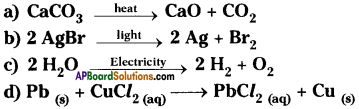a) It is a decomposition reaction carried out in the presence of heat. So it is thermal decomposition reaction.
b) It is a decomposition reaction carried out in the presence of light. So it is photochemical reaction.
c) It is a decomposition taking place in the presence of electricity. So it is electrolysis reaction.
d) Lead is more reactive than copper. So lead displaces copper from salt solution. So the reaction is chemical displacement reaction.

Question 29.
Ramu told Ravi that all material made of iron and its alloys are rusted when exposed to air. How do you correct him by asking suitable questions?

1. What are the material we will generally use in our cooking utensils?
2.  What are the material used for surgical equipments?
3. What happens when stainless steel vessels are exposed to air?

Question 30.
What are the gases released when lead nitrate is heated and how do you identify those gases?
When lead nitrate is heated it decomposes into lead oxide, nitrogen dioxide and oxygen.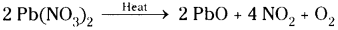Nitrogen diox-ide is identified by its reddish brown co|our. Oxygen makes the burning splinter to burn brightly when it is placed in test tube containing oxygen.

Question 31.
Write some chemical reactions occurring in our daily life.

1. Souring of milk
2. Formation of curd from milk
3. Cooking of food
4. Digestion of food in our body
5. Fermentation of grapes
6. Rusting of iron
7. Burning of fuels
8. Burning of candle wax
9. Ripening of fruits.

Question 32.
If 40 gm of methane is burnt, then how much amount of CO2 is released ?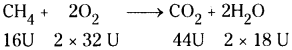When 16 g of methane is burnt it releases 44 g of CO2. The amount of CO2 released when 40 g methane burnt 44
= $$\frac{44}{16}$$ × 40=110g

Question 33.
Calculate the amount of calcium oxide formed when 2 kg of calcium carbonate is decomposed. (The atomic masses of Ca = 40 U, C = 12 U, O = 16 U).
The balanced equation is
CaCO3(s) → CaO(s) + CO2(g)
(40 + 12 + 3 x 16) U → (40 + 16) U + (12 + 2 x 16) U
100 U → 56 U + 44 U
100 g → 56g + 44g
100 g calcium carbonate on decomposition produces 56 g of calcium oxide.
Amount of calcium oxide formed due to decomposition of 2 kg of calcium carbonate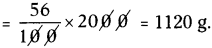Question 34.
A solution of common salt when added to silver nitrate solution yields a precipitate of silver chloride (0.28 g). Find the mass of sodium chloride in the solution and also the mass of sodium nitrate formed.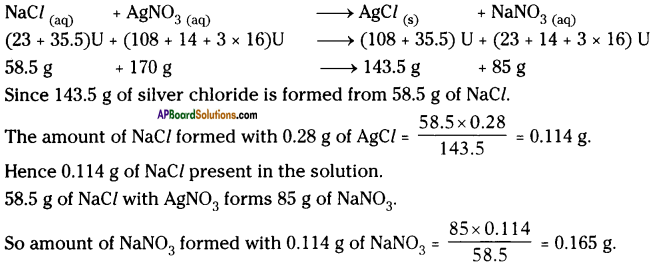Question 35.
0.29 g of hydrocarbon when burnt completely in oxygen produces 448 ml of carbon dioxide at STP. From the information, calculate the
i) mass of carbon dioxide formed
ii) mass of element carbon in carbon dioxide
iii) mass of hydrogen in hydrocarbon.
i) Gram molecular weight of carbon dioxide = 12 + 2 × 16g = 44 g.
22.4 litres of CO2 at STP weighs 44 g.

The weight of 448 ml of CO2 at STP = $$\frac{44}{22400}$$ × 448 = 0.88 g

ii) 44g of CO2 contains 12 g of carbon.
The amount of carbon present in 0.88 g of CO2 = $$\frac{12}{44}$$ × 0.88 = 0.24 g

iii) Weight of hydrogen = Weight of hydrocarbon – Weight of carbon
= 0.29 – 0.24 = 0.05 g

Question 36.
Calculate the weights of carbon dioxide and water that will be obtained by completely burning 0.25 g of an organic compound having molecular formula C4H4O4.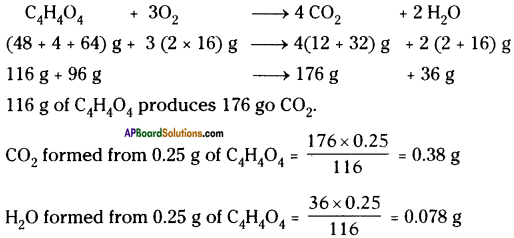Question 37.
What weight of sulphuric acid will be required to completely dissolve 3g of magnesium carbonate? Calculate the volume of carbon dioxide evolved at STP.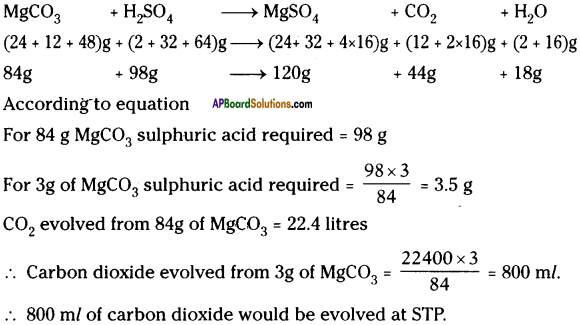Question 38.
If 16.4 g of calcium nitrate is heated : (a) Calculate the volume of Nitrogen dioxide obtained at STP and (b) The mass of calcium oxide obtained.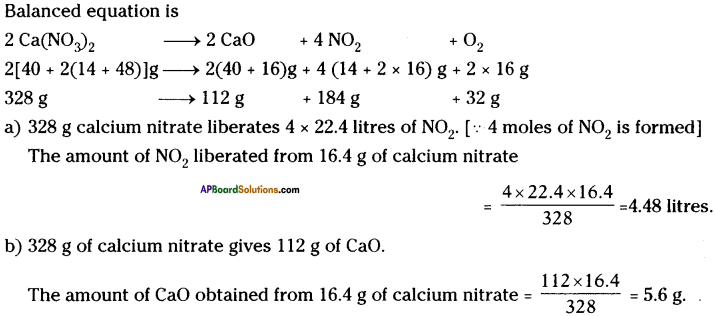Question 39.
Give some examples for corroded and non-corroded metals and give the reasons for non-corrosion of metals.
Examples to corroded metals :

1. Iron
2. Copper
3. Silver

Reactions:
4Fe + 3O2 → 2Fe2O3
2Cu + O2 → 2CuO
4Ag + 2H2S + O2 → 2Ag2S + 2H2O

Exmples to non-corroded metals :

1. Gold
2. Platinum
3. Brass
4. Bronze
5. Steel

Reasons:

1. Gold and platinum metals do not react with oxygen and have resistance to corrosion.
2. Brass, bronze and steel are alloys. So they have a quality of resistance to corrosion.Question 40.
Iron is a corroded metal. Through alloying we can prevent corrosion. Justify.

• Alloying is a very good method of improving properties of metal.
• Generally pure form of iron is very soft and stretches easily when hot.
• When iron is mixed with carbon, nickel and chromium, an alloy stainless steel is obtained.
• The stainless steel is hard and does not rust.
• So, through alloying iron we can prevent corrosion.

Question 41.
“Through alloying corrosion can be prevented.” For the justification pose some questions.

1. What is alloying?
2. How are alloys prepared?
3. What are the qualities of alloys?
4. Which quantity prevents the corrosion in alloys?
5. Can we prevent corrosion by alloying a metal ?

9th Class Physical Science6th Lesson Chemical Reactions and Equations 4 Marks Important Questions and Answers

Question 1.
Write the balanced chemical reaction for the following and identify the type of reaction in each case.
A) Magnesium(s) + Iodine(g) → Magnesium iodide(s)
B) Zinc(s) + Hydrochloric acid(aq) → Zinc chloride(aq) + Hydrogen(g)
A) Magnesium(s) + Iodine(g) → Magnesium iodide(s)
Mg + I2 → Mgl2. This reaction is chemical combination.

B) Zinc(s) + Hydrochloric acid(aq) → Zinc chloride(aq) + Hydrogen(g)
Zn + 2HCl → ZnCl2 + H2
This reaction is chemical displacement.Question 2.
Write an activity to each of the following chemical reaction.
A) Photo chemical reaction
B) Chemical displacement reaction.
A) Photo chemical reaction :
1) Take a pinch of Silver Bromide in a watch glass and it in the presence of sunlight.
2) Silver Bromide decomposes to silver and Bromine in sunlight.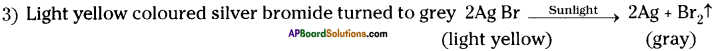B) Chemical displacement:

1. Take a small quantity of zinc dust in a conical flask and add some drops of dilute hydrochloric acid slowly.
2. Immediately we can observe the gas bubbles coming out from the solution.
3. This is because zinc reacts with dilute hydrochloric acid and liberates hydrogen gas.
Zn + 2HCl → ZnCl2 + H2
4. In this reaction the element zinc has displaced hydrogen from hydrochloric acid.

Question 3.
Why should we balance a chemical equation ? Take any one chemical equation and explain the procedure of balancing it.
Chemical reactions obey law of conservation of mass. So the total number of atoms of each elements in the reactants must be equal to the total number of atoms of each element in the

products. So we should have to balance chemical equation.
Eg : H2 + O2 → H2O

Step -1 : Unbalanced equation = H2 + O2 → H2O
Step – 2 : Compare no.of atoms of each element on both sides.

 Atom No. of atoms in L.H.S No. of atoms in R.H.S H 2 (in H2) 2 (in H2O) 0 2 (in O2) 1 (in H2O)

No.of 0 atoms balancing – H2 + O2 → 2H2O
No.of H atoms balancing – 2H2 + O2 → 2H2O

Step – 3 : The above equation is balanced and write the coefficients in the smallest ratio. 2H2 + O2 → 2H2O

Step – 4 : Verify above equation for balancing of atoms each element on both sides. Hence the equation is balanced.
∴ 2H2 + O2 → 2H2O

Question 4.
Balance the following chemical equations.
i) Zn(s) + Ag NO3(aq) → Zn (NO3)2(aq) + Ag(s)
ii) Fe2O3(s) + C(s) → Fe(s) + CO2(g)
iii) Ag(s) + H2S(g) → Ag2S(s) + H2O(l)
iv) Cu(s) + O2(g) → CuO(g)
i) Zn(s) + Ag NO3(aq) → Zn (NO3)2(aq) + Ag(s)
Balanced equation : Zn(s) + 2 AgNO3(aq) → Zn (NO3)2(aq) + 2Ag(s)

ii) Fe2O3(s) + C(s) → Fe(s) + CO2(g)
Balanced equation : 2Fe2O3(s) + 3C(s) → 4Fe(s) + 3CO2(g)

iii) Ag(s) + H2S(g) → Ag2S(s) + H2O(l)
Balanced equation :
We cannot balance the equation because atom ‘O’ does not exist in the reactants.

iv) Cu(s) + O2(g) → CuO(s)
Balanced equation :
2Cu(s) + O2(g) → 2CuO(s)

Question 5.
Write the equation for the reaction of zinc with hydrochloric acid and balance the equation. Find out the number of molecules of hydrogen gas produced in this reaction, when 1 mole of HCl completely reacts at S.T.P.
[Gram molar volume is 22.4 liters at S.T.P., Avogadro’s number is 6.023 × 1023]
Zn + 2HCl → ZnCl2 + H2

• In the reaction 2 moles of HC/ produce 1 mole of H2.
• If 1 mole of HCl participate in reaction, Vi mole of hydrogen will be produced.
• 1 mole of H2 gas contains 6.023 × 1023 molecules at STP.
Number of molecules in ½ mole of H2 gas = 6.023 × 1023 × ½ = 3.011 × 1023

Question 6.
Write the examples for chemical combination.
1) Magnesium burns in oxygen to form magnesium oxide.
2 Mg + O2 → 2 MgO

2) When coal is burnt in oxygen, carbon dioxide is produced.
C + O2 → CO2 + Q (cheat energy)

3) Slaked lime is prepared by adding water to quick lime.
CaO + H2O → Ca(OH)2 + Q (heat energy)

4) When hydrogen reacts with oxygen, it gives water.
H2 + O2 → 2 H2O

5) Hydrochloric acid is obtained by adding hydrogen to chlorine.
H2 + Cl2 → 2 HCl

6) Magnesium reacts with iodine to magnesium iodide.
Mg + l2 → Mgl2

7) Sodium reacts with chlorine to form sodium chloride.
2 Na +Cl2 → 2 NaCl

8) Iron reacts with oxygen to form haematite.
Fe + 3O2 → 2 Fe2O3

Question 7.
Write examples for chemical decomposition reaction.
1) Calcium carbonate on heating decomposes to calcium oxide and carbon dioxide.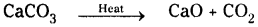2) On electrolysis, water decomposes to water and hydrogen.
2 H2O → 2 H2 + O2

3) Silver bromide decomposes to silver and bromine in sunlight.
2 AgBr → 2 Ag + Br2

4) Silver chloride decomposes to silver and chlorine.
2 AgCl → 2 Ag + Cl2

5) Glucose decomposes to ethanol and carbon dioxide.
C6H12O6 → 2 C2H5OH + 2 CO2

6) Sodium bicarbonate decomposes to sodium carbonate, water and carbon dioxide.
2 NaHCO3 + Heat → Na2CO3 + H2O + CO2

7) On heating lead nitrate decomposes to lead oxide, oxygen and nitrogen dioxide.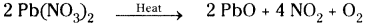Question 8.
Write the examples for chemical displacement reaction.
1) Zinc pieces react with dilute hydrochloric acid and liberate hydrogen gas
Zn + 2 HCl → ZnCl2 + H2

2) Iron reacts copper sulphate to form iron sulphate and copper.
Fe + CuSO4 → FeSO4 + Cu

3) Zinc is mixed with silver nitrate to form zinc nitrate and silver.
Zn + 2 AgNO3 → Zn(NO3)2 + 2Ag

4) Lead reacts with copper chloride to form lead chloride and copper.
Pb + CuCl2 → PbCl2 + Cu

5) Sodium reacts with water to form sodium hydroxide and hydrogen.
2 Na + 2H2O → 2 NaOH + H2

6) Aluminium reacts with copper chloride to form aluminium chloride and copper.
2 Al + 3 CuCl2 → 2 AlCl3 + 3 Cu

7) Zinc reacts with sulphuric acid to form zinc sulphate and hydrogen.
Zn + H2SO4 → ZnSO4 + H2Question 9.
Write examples for chemical double displacement reaction.
1) Sodium sulphate solution on mixing with barium chloride solution forms a white precipitate of barium sulphate and soluble sodium chloride.
Na2SO4 + BaCl2 → BaSO4 + 2 NaCl

2) Sodium hydroxide reacts with hydrochloric acid to form sodium chloride and water.
NaOH + HCl → NaCl + H2O

3) Sodium chloride spontaneously combines with silver nitrate in solution giving silver chloride precipitate.
NaCl + AgNO3 → AgCl + NaNO3

4) Mix lead nitrate solution and potassium iodide solution to form a yellow precipitate of lead iodide and potassium nitrate.
Pb(NO3)2 + 2Kl → PbI2 + 2KNO3

5) Calcium hydroxide reacts with nitric acid to form water and calcium nitrate.
Ca(OH)2 + 2 HNO3 → 2H2O + Ca(NO3)2

6) Magnesium chloride reacts with potassium hydroxide to form magnesium hydroxide and potassium chloride.
MgCl2 + 2 KOH → Mg(OH)2 + 2 KCl

Question 10.
Balance the following equations.
1) Al(OH)3 → Al2O3 + H2O
2) NH3 + CuO → Cu + N2 + H2O
3) Al2(SO4)3 + NaOH → Al(OH)3 + Na2SO4
4) HNO3 + Ca(OH)2 → Ca(NO3)2 + H2O
5) NaOH + H2SO4 → Na2SO4 + H2O
6) BaCl2 + H2SO4 → BaSO4 + HCl

1. 2 Al(OH)3 → Al2O3 + 3 H2O
2. 2 NH3 + 3 CuO → 3 Cu + N2 + 3 H2O
3. Al2(SO)3 + 6 NaOH → 2 Al(OH)3 + 3 Na2SO4
4. 2 HNO3 + Ca(OH)2 → Ca(NO3)2 + 2 H2O
5. 2 NaOH + H2SO4 → Na2SO4 + 2 H2O
6. BaCl2 + H2SO4 → BaSO4 + 2 HClQuestion 11.
How many types of chemical reactions are there? Explain with examples.
There are four types of chemical reactions. They are :
i) Chemical combination :
A chemical reaction in which two or more substances combine together to form a new substance is called chemical combination.
e.g.: 2H2 + O2 → 2H2O
CaO + H2O → Ca(OH)2

ii) Chemical decomposition :
The reaction in which a compound breaks up into two or more simpler substances are known as decomposition reaction. These reactions are generally carried out by means of heat, light, electricity or catalysts.
e.g.: CaCO3 → CaO + CO2
2Pb(NO3)2 → 2 PbO + 4NO2 + O2

iii) Chemical displacement:
The chemical reaction in which one element takes the place of another element in a compound is called displacement reaction.
In these reactions, an atom or group of atoms in a molecule is replaced by another atom or a group of atoms.
e.g.: Zn + 2HCl → ZnCl2 + H2
Zn + CuSO4 → ZnSO4 + Cu

iv) Chemical double displacement: The reaction in which two compounds react to form two other compounds by mutual exchange of their ions is called double displacement reaction.
e.g.: NaOH(aq) + HCl(aq) → NaCl(aq) + H2O(l)
NaNO3(aq) + AgCl(aq) → AgNO3(s) + NaCl(aq)

Question 12.
Balance the following chemical equations.
a) Na2SO4 + BaCl2 → BaSO4 + NaCl
b) Al4C3 + H2O → CH4 + Al(OH)3
c) Pb(NO3)2 → PbO + NO2 + O2
d) Fe2O3 + Al → Al2O3 + Fe
a) Na2SO4 + BaCl2 → BaSO4 + 2NaCl
b) Al4C3 + 12H2O → 3CH4 + 4Al(OH)3
c) 2Pb(NO3)2 → 2PbO + 4NO2 + O2
d) Fe2O3 + 2Al → Al2O3 + 2FeQuestion 13.
How can we make a chemical equation information?
1. Expressing the physical state :
The different states, i.e. gaseous, liquid and solid states are represented by the notations (g), (l) and (s) respectively. If the substance is present as a solution in water the word aqueous is written.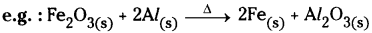2. Expressing the heat changes :
Q is heat energy which is shown with plus ’+’ sign on product side for exothermic reactions and minus sign on product side for endothermic reactions.
e.g.: a) C(s) + O2(g) → CO2(g) + Q (exothermic reaction)
N2(g) + O2(g) → 2NO(g) ” Q (endothermic reaction)

3. Expressing the gas evolved :
If a gas evolved in a reaction, it is denoted by an upward arrow ↑ or (g).
Zn(s) + H2SO4(aq) → ZnSO4(aq) + H2(g)

4. Expressing precipitate formed: If a precipitate is formed in the reaction, it is denoted by downward arrow ↓.
AgNO3(aq) + NaCl(aq) → AgCl(s) ↓ + NaNO3(aq)

Question 14.
Give daily life examples of oxidation.
Daily life examples :

1. Combustion of fuels.
2. Corrosion of metals.
3. Change of colour of fruits like apples, bananas, when they are cut.
4. Burning of crackers.
5. Rancidity of food material.
6. During rainy season the power supply to our home from the electric pole will be interrupted due to formation of metal oxide layer on the electric wire.
7. Rising of dough with yeast depends on oxidation of sugars to carbon dioxide and water.
8. Bleaching of coloured objects using moist chlorine.
9. Respiration.

Question 15.
What is the information giyen by balanced chemical equation?

• A chemical equation gives information about the reactants and products through their symbols and formulae.
• It gives the ratio of molecules of reactants and products.
• As molecular masses are expressed in unified masses, the relative masses of reactants and products are known from the equation.
• If the masses are expressed in grams, then the equation also gives the molar ratios of reactants and products.
• If gases are involved, we can equate the masses to their volumes.
• Using molar mass and Avagadro’s number we can calculate the number of molecules and atoms of different substances from the equation.

Question 16.
Write the balanced equation and identify the type of reaction.
1) Magnesium Hydroxide(aq) + Nitric Acid(aq) → Magnesium Nitrate(aq) + Water(l)
2) Magnesium(s) + Carbon Monoxide(g) → Magnesium Oxide(s) + Carbon(g)
3) Barium Chloride(aq) + Sodium Sulphate(aq) → Barium Sulphate(s) + Sodium Chloride(aq)
4) Sodium Nitrate(s) → Sodium Nitrite(s) + Oxygen(g)
1) Mg(OH)2(aq) + 2 HNO3(aq) → Mg(NO3)2(aq) + 2 H2O(l)
It is both double displacement and neutralisation reaction.

2) Mg(s) + CO(g) → MgO(s) + C(s)
It is a redox reaction in which magnesium is oxidised and carbon monoxide is reduced.

3) BaCl2(aq) + Na2SO4(aq) → BaSO4(s) ↓ + 2 NaCl(aq)
It is both precipitation and double displacement reaction.

4) 2 NaNO3(s) → 2 NaNO2(s) + O2(g)
It is both endothermic and decomposition reaction.Question 17.
We write symbol of water as H2O. State why we should not write it as HO2.
1) The hydrogen atom has only T electron in its outermost shell, so it needs 1 more electron to achieve the stability. ‘2’ electrons are required to get inert gas electronic configuration.

2) The oxygen atom has ‘6’ electrons in its outermost shell and it needs ‘2’ more electrons to compare the stability. ‘8’ electron arrangement of inert gas is neon.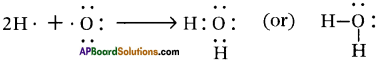In the water molecule, central oxygen atom has two pairs of unshared electrons which have not been utilised in the formation of bonds.

So, we write symbol of water as H20 and we should not write it as HO2.

Question 18.
Latha took some quantity of powder of a substance in a test tube. Heated it with spirit lamp. A gas was liberated. She sent the gas into another test tube. The colour of solution in the second test tube turned into milk white.
a) Which substance was heated?
b) Which gas was liberated?
c) What was the solution taken in second test tube?
d) Which type of chemical reactions involved the experiment?
a) The substance is calcium carbonate.
b) The gas liberated is carbon dioxide.
c) The solution taken in the second test tube was solution of slaked lime.
d) Two types of reactions took place in this experiment, i.e. decomposition and double displacement.
CaCO3(s) → CaO(s) . CO2
Ca(OH)2(aq) + CO2(g) → CaCO3(s) + H2O

Question 19.
A light yellow colour substance (some quantity) on a watch glass is put in the sunlight. It changes into grey colour substance.
a) What is the light yellow colour substance?
b) What is the grey colour substance?
c) Which type of chemical reaction it is?
d) Write the chemical equation for the reaction.
a) The light yellow colour substance is silver bromide.
b) The grey colour substance is silver.
c) The type of chemical reaction is photochemical reaction.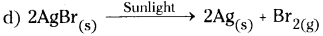Question 20.
Heat is liberated in the reactions where water is added to calcium oxide and hydrochloric acid added to zinc pieces.
Rarnu says that they are same type of chemical reactions. Eswar’s opinion is that the reactions are not similar. What is the basis of Eswar’s opinion ? Write equations for the above reaction.
Eswar’s thinking is correct. Although both are exothermic reactions, the type of chemical reactions is different.
Case (i) : When water is added to calcium oxide it forms calcium hydroxide. It is an example for combination reaction.
CaO(s) + H2O → Ca(OH)2(aq)

Case (ii) : When hydrochloric acid is added to zinc pieces it would liberate hydrogen gas which is an example for displacement reaction.
Zn(s) + HCl(aq) → ZnCl2(aq) + H2(g)Question 21.
We see many combustion and oxidation reactions in our daily life. Among them every combustion reaction is an oxidation reaction. But not all the oxidation reactions are combustion reactions. Do you agree or disagree with the statement? Explain with proper reasons.
1) Yes, I agree with the statement because combustion reaction is nothing but burning of a substance in the presence of oxygen so it is an oxidation reaction. So every combustion reaction is an oxidation reaction.

2) Whereas some reactions which do not require burning still they are oxidation reactions.
Eg :

1. Corrosion of metals.
2. Change of colour of fruits like apples, bananas when they are cut.
3. Rancidity of food materials.
4. Respiration.
5. Bleaching of coloured objects using moist chlorine.
So all oxidation reactions are not combustion reactions.

Question 22.
Explain the following :
1) What happens when iron filings are added to zinc sulphate solution?
2) What happens when solid silver nitrate is added to solid sodium chloride?
1) No reaction takes place because zinc is more reactive than iron. So iron cannot displace zinc from its salt solution.

2) No reaction takes place because in solid state silver nitrate as well as sodium chloride is unable to dissociate into constituent ions. So exchange of ions is not possible.

Question 23.
Why is steel not used for surgical equipment and what is the material used for surgical equipment? Why is that material used for surgical equipment?
1) Steel undergoes rusting when It exposed to air. So it is not useful for preparation of surgical equipment. If we use it in surgical equipment it may cause septic of wound.

2) The material used for surgical equipment is stainless steel which is an alloy of iron, carbon, nickel and chromium. Chromium does not easily react with oxygen. So, addition of chromium makes the stainless steel free from rusting. Therefore it is used in surgical equipment.Question 24.
Give reasons for the following.
1) Why do we add salt to water during electrolysis of water?
2) Why does hydrogen gas put off the burning splinter and it burns with blue flame?
3) Why does carbon dioxide turn lime water milky?
1) Pure water is a bad conductor of electricity. By adding a small amount of salt the solution becomes conductor of electricity.

2) Hydrogen does not support combustion. So it puts off the burning splinter and also it is combustible. So burns with blue flame.

3) When we pass carbon dioxide through lime water it turns into milky because lime water (calcium hydroxide) reacts with carbon dioxide and forms a white milky substance, i.e. calcium carbonate.

Question 25.
A student was given the following substances and was asked to show types of chemical reactions through experiment. Write how he would have done that. Copper sulphate solution, barium chloride solution, ferrous sulphate crystals, iron nails, calcium oxide, water.
Given chemicals are CuSO4 solution, BaSO4 solution, iron nails (Fe), Ferrous sulphate (FeSO4), Calcium oxide (CaO) and water (H2O).

i) Chemical combination :
Chemicals chosen : CaO, H2O
CaO + H2O → Ca(OH)2

When we add water to calcium oxide it produces Calcium hydroxide. This is an example for chemical combination.

ii) Chemical decomposition:
Chemicals chosen : Ferrous sulphate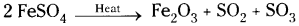When we heat FeSO4 it dissociates into Ferric oxide, Sulphur dioxide and Sulphur trioxide respectively.

iii) Chemical displacement :
Chemicals chosen : Iron nail – CuSO4
Fe + CuSO4 → FeSO4 + Cu ↓

When iron nail is placed in CuSO4 solution, the solution turns into light green due to formation of FeSO4 and reddish brown deposit of Copper.

iv) Double displacement:
Chemicals chosen : CuSO4 solution and BaCl2 solution.
CuSO4(aq) + BaCl2(aq) → BaSO4(s) ↓ + CuCl2(s)

When CuSO4 is mixed with BaCl2 solution it forms white precipitate of BaSO4 and aqueous solution of Copper (II) Chloride.

This is an example for double displacement reaction.Question 26.
Take two beakers and prepare lead nitrate aqueous solution and potassium iodide aqueous solutions. What are the colours of the solutions? Now mix them in another beaker. What happens? What type of chemical reaction it is? What are products?
The colours of the solutions are white or colourless. When lead nitrate solution is mixed with potassium iodide solution we will get yellow precipitate of lead iodide. This is double displacement reaction. The products are lead iodide and potassium nitrate.
Pb(NO3)2(aq) + 2Kl(aq) → Pbl2(s) + 2KNO3(aq)

Question 27.
Observe the following equation which shows the action of heat on Calcium Nitrate
2 Ca(NO3)2 → 2 CaO + 4 NO2 + O2
a) How many moles of NO2 are formed when a mole of 2 Ca(NO3)2 is decomposed?
b) What is the volume of NO2 produced when 164 gm of Ca(NO3)2 is heated at constant temperature and pressure?
c) Calculate the mass of Calcium Oxide formed when 82 gm of Ca(NO3)2 is heated.
d) What is the quantity of Ca(NO3)2 required to produce 5 moles of gaseous products?
Given balanced equation is
a) From the balanced equation 2 moles of Ca(NO3)2 releases 4 moles of NO2.
b) Molecular weight of Ca(NO3)2 and CaO respectively are 164 and 56.
From the equation at STP 2 × 164 g. of Ca(NO3)2 releases 4 × 22.4 litres of NO2.
At similar conditions 164 g. of Ca(NO3)2 releasing NO2 in litres is
= $$\frac{164}{2 \times 164}$$ × 22.4 × 4 = 2 × 22.4 = 44.8 liters.

c) From the balanced equation 164 g. of Ca(NO3)2 decomposes and forms 112 g. of CaO. Similarly 82 g. of Ca(NO3)2 decomposes and forms
$$\frac{82}{164}$$ × 112 = 56 g. of CaO.

d) From the above balanced equation 2 moles of Ca(NO3)2 releases 5 moles of gaseous products.
∴ The mass of Ca(NO3)2 required is 2 × 164 = 328 g.Question 28.
Zn + HCl → ZnCl2 + H2
Calculate amount of zinc required to release 500g of hydrogen. (Zn = 65 U, H = 1 U, Cl = 35.5 U are the atomic masses).
The balanced chemical equation is
Zn + 2 HCl → ZnCl2 + H2
65 U + (2 × 36.5) U → (65 + 2 × 35.5) U + 2 U
65 g + 73 g → 136 g + 2g
As per the balanced equation
65 g Zinc is reacting with hydrochloric acid to produce 2g of Hydrogen.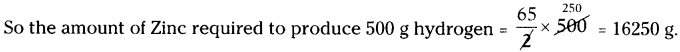Question 29.
Calculate the volume, mass and number of molecules of carbon dioxide when 104 g of acetylene (C2H2) burnt in air. (Atomic masses of C = 12 U, H = 1 U, O = 16 U).
The balanced chemical equation is
2C2H2 + 5O2 → 4CO2 + 2H2O
2 × (2 × 12U + 2 × lU) + 5 × (2 × 16U) → 4 × (12U + 2 × 16U) + 2(2 × 1U + 16U)
52 g + 160g → 176 g + 36 g
52 g of acetylene when burnt in air produces 176 g carbon dioxide.
The amount of carbon dioxide produced when 104 g acetylene burnt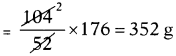At S.T.P. 1 gram molar mass of any gas occupies 22.4 litres.
So 44g carbon dioxide occupies 22.4 litres volume.
The volume occupied by 352 g carbon dioxide = $$\frac{352}{44}$$ × 22.4 = 8 × 22.4 = 179.2 litres.
44 g of carbon dioxide i.e., 1 mole of CO2 contains 6.02 × 1023 molecules. So the number of molecules present in 352 g of carbon dioxide
352
= $$\frac{352}{44}$$ × 6.02 × 1023 = 8 × 6.02 × 1023
= 4.816 × 1024 molecules.